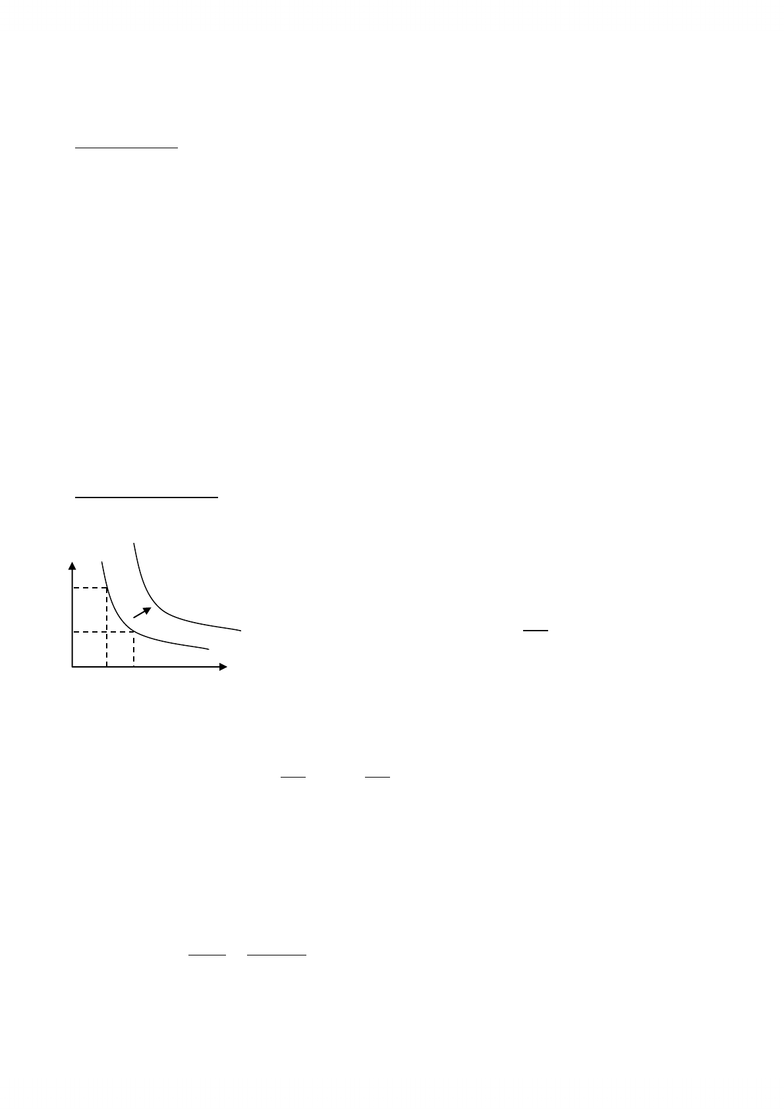# ECO206Y1 Lecture Notes - Indifference Curve, Utility, Lincoln Near-Earth Asteroid Research

60 views3 pages
School
Department
Course
ProfessorTopic 2 Utility & Indifference Curve
(Lecture 2 Sept 21st)
Utility Function: CU = u(x1, x2, x3, , xn)
Math representation of preference ordering:
a. X>Y U(x)>U(y)
b. XY U(x)=U(y)
* numbers in the utility function are ordinal not cardinal*
From the utility function, we only know the order of the preference (whether X is before Y),
not the strength of the preference (whether the utility of X is 3 times greater than Y)
* These utility functions are only unique to a positive monotonic transformation.
e.g. V[U(x) = [U(X)]2
= 3 + 2U(X)
= 5 + lnU(X)
All these are examples of positive monotonic transformation of the Utility function;
The preference order is the same;
Indifference Set/curve
1. Set of commodity bundles that satisfies U(x1, x2) = Uo (some level of utility)
x2
x1
2. Total Differentiate U(x1, x2) = Uo


   

   
MU of x1 MU of x2
*MU of x1: the utility one gets from consuming one more unit of x1, holding x2 constant.
Implication:

 
 
Measure the rate at which the consumer would be willing to substitute one good for another
-- Indifference curve pass to the right indicate higher utility
(assume more is better)
-- Slope of the indifference curve: 

Unlock document

This preview shows page 1 of the document.
Unlock all 3 pages and 3 million more documents.

# Get access

\$10 USD/m
Billed \$120 USD annually
Homework Help
Class Notes
Textbook Notes